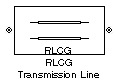# RLCG Transmission Line

Model RLCG transmission line

• Library:
• RF Blockset / Equivalent Baseband / Transmission Lines

•## Description

The RLCG Transmission Line block models the RLCG transmission line described in the block dialog box in terms of its frequency-dependent resistance, inductance, capacitance, and conductance. The transmission line, which can be lossy or lossless, is treated as a two-port linear network.where z′ = z + Δz.

To learn how you can use RLCG Transmission Line block to create baseband equivalent model, see Create Complex Baseband-Equivalent Model.

## Parameters

expand all

### Main

Vector of resistance values in ohms per meter, specified as a scalar in meters.

Vector of inductance values in henries per meter, specified as a scalar in meters.

Vector of capacitance values in farads per meter, specified as a scalar in meters

Vector of conductance values in siemens per meter.

Vector of frequency values at which the resistance, inductance, capacitance, and conductance values are known.

Specify the interpolation method, the block uses to calculate the parameter values at the modeling frequencies.

Physical length of the transmission line, specified in meters.

The block enables you to model the transmission line as a stub or as a stubless line.

#### Stubless transmission Line

• `Not a stub`Not a stub

If you model a coaxial transmission line as stubless line, the Coaxial Transmission Line block first calculates the ABCD-parameters at each frequency contained in the modeling frequencies vector. It then uses the `abcd2s` function to convert the ABCD-parameters to S-parameters. For more information, see Stub Mode - Not a Stub.

#### Shunt Transmission Line

• `Shunt`—This parameter provides a two-port network that consists of a stub transmission line that you can terminate with either a short circuit or an open circuit as shown in these diagrams.Zin is the input impedance of the shunt circuit. The ABCD-parameters for the shunt stub are calculated as

`$\begin{array}{c}A=1\\ B=0\\ C=1/{Z}_{in}\\ D=1\end{array}$`

#### Series Transmission Line

• `Series`—This mode parameter provides a two-port network that consists of a series transmission line that you can terminate with either a short circuit or an open circuit as show in these diagrams.Zin is the input impedance of the series circuit. The ABCD-parameters for the series stub are calculated as

`$\begin{array}{c}A=1\\ B={Z}_{in}\\ C=0\\ D=1\end{array}$`

Stub termination for stub modes `Shunt` and `Series`. Choices are `Open` or `Short`

#### Dependencies

To enable this parameter, select `Shunt` or `Series` in Stub mode

### Visualization

When Source of frequency data is `User-specified`, specify as a vector of frequencies in the Frequency data parameter.

Frequency data range, specified as a vector in hertz.

#### Dependencies

To set this parameter, first select `User-specified` in Source of amplifier gain. This selection activates the Visualization Tab which contains Source of frequency data

Reference impedance of the coaxial transmission line, specified as a scalar in ohms.

Type of data plot that you want to produce with your data specified as :

• `X-Y plane` — Generate a Cartesian plot of your data versus frequency. To create linear, semi-log, or log-log plots, set the Y scale and X scale accordingly.

• `Composite data`—The composite data plot automatically generates four separate plots in one figure window, showing the frequency dependence of several parameters.

• `Polar plane` — Generate a polar plot of your data. The block plots only the range of data corresponding to the specified frequencies.

• `Z Smith chart`, ```Y Smith chart```, and ```ZY Smith chart``` — Generate a Smith® chart of your data. The block plots only the range of data corresponding to the specified frequencies.

Type of parameters to plot, specified as one of the following `S11`, `S12`, `S21`, `S22`, `GroupDelay`, `GammaIn`, `GammaOut`, `VSWRIn`, `VSWROut`, `OIP3`, `IIP3`, `TF1`, `TF2`, `TF3`, `Gt`, `Ga`, `Gp`, `Gmag`, `Gmsg`, `GammaMS`, `GammaML`, `K`, `Delta`, `Mu` or `MuPrime`. When noise is spectral `NF`, `NFactor` and `NTemp` plotting is possible.

Type of parameters to plot, specified as one of the following `S11`, `S12`, `S21`, `S22`, `GroupDelay`, `GammaIn`, `GammaOut`, `VSWRIn`, `VSWROut`, `OIP3`, `IIP3`, `TF1`, `TF2`, `TF3`, `Gt`, `Ga`, `Gp`, `Gmag`, `Gmsg`, `GammaMS`, `GammaML`, `K`, `Delta`, `Mu` or `MuPrime`. When noise is spectral `NF`, `NFactor` and `NTemp` plotting is possible.

Plot format, specified as one of the following ```Magnitude (decibels)```, `Angle (degrees)`, `Real`, or `Imaginary`.

Plot format, specified as one of the following ```Magnitude (decibels)```, `Angle(degrees)`, `Real`, or `Imaginary`.

Parameter, specified as `Freq`. This parameter determines the data for x-axes on the X-Y plane plot.

Plot format, specified as one of the following `Hz`, `Auto`, `KHz`, `MHz`, `GHz` or `THz`.

Y-axis scale, specified as `Linear` or `Log`.

X-axis scale, specified as `Linear` or `Log`.

Plot the specified data using the plot button.